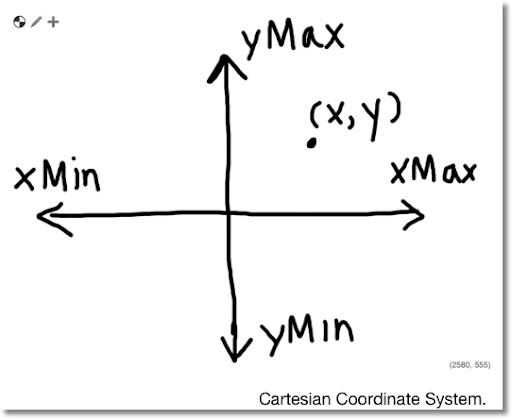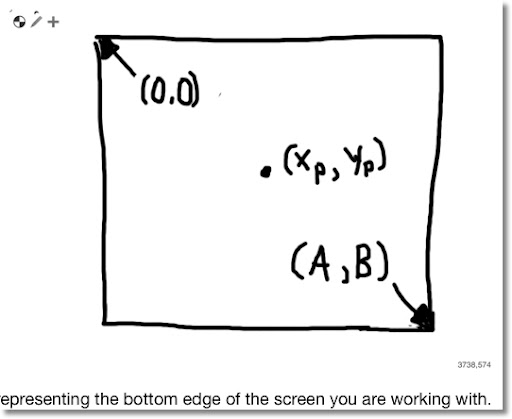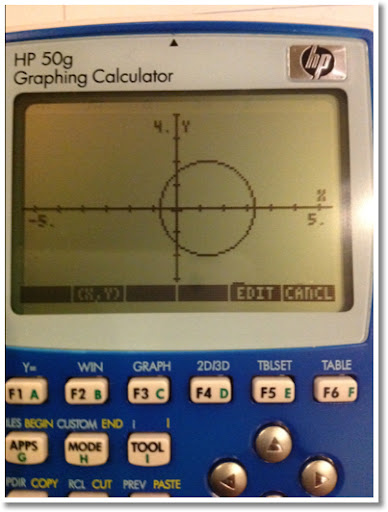## Thursday, September 13, 2012

### Cartesian Coordinates to Pixel (Screen) Coordinates

Converting Cartesian Coordinates to Screen Coordinates

When working with computer or calculator graphics, sometimes we have to work with screen coordinates. The screen coordinate system has the following:

1. The upper corner pixel (point) is (0,0).
2. The lower corner pixel is (A, B).
3. The x axis increases in the right direction.
4. The y axis increases in the down direction, the opposite direction of the Cartesian plane.In order to to convert a point (x, y) in the Cartesian coordinates to point (xp, yp) in Screen coordinates, first observe the following:

1. Picture the plane you are working with as a screen. If you are working with a calculator or computer, this is fairly easy.
2. Let Xmin be the least-valued x of the screen (left edge) and Xmax be the most-valued x (right edge). Similarly, let Ymin be the least-valued y of the screen (bottom) and Ymax be the most-valued y of the screen (top).

You can check with the window settings on a graphing calculator to verify Xmax, Xmin, Ymax, and Ymin.

For the Hewlett Packard 28 series, 48 series and 50g, the variables XRNG and YRNG list the screen's dimensions, {Xmin, Xmax} for XRNG and {Ymin, Ymax} for YRNG.

To transform Cartesian coordinates to screen coordinates, we can use transformation matrices for scaling and translation. The general formshow the transformation from coordinates (x, y) to (x', y') where:

s_x = scale of the new x axis. If s_x < 0, the x point is reflected with respective to the x axis.

s_y = scale of the new y axis. If s_y < 0, the y point is reflected with respected to the y axis. In going from Cartesian coordinates to screen coordinates, s_y < 0.

x_s = Is the translation (shift to the new center) in the x-direction. If x_s > 0, the new center is to the right of the original center. Similarly, if x_s < 0, the new center is to the left of the original center.

y_s = Is the translation (shift to the new center) in the y-direction. If y_s > 0, the new center is to the above the original center. Similarly, if y_s < 0, the new center is to the below the original center.

For the transformation from Cartesian coordinates to screen coordinates:

s_x = A / (Xmax - Xmin)

s_y = -B / (Ymax - Ymin)

Since the new center will be at (Xmin, Ymax):

x_s = -Xmin

y_s = -Ymax

Therefore by matrix multiplication, with x' = xp and y' = yp:

xp = (x - Xmin) * A / (Xmax - Xmin)

yp = (y - Ymax) * -B / (Ymax - Ymin)

On the HP 48 series and 50g, the translation from Cartesian to screen coordinates can be accomplished with the C→PX function.

Example:

Using the following window with settings Xmin = -5, Xmax = 5, Ymin = -4, and Ymax = 4, transform the Cartesian coordinate (1, 0) to screen coordinates. The screen has pixel size 130 × 79.

In this case, A = 130 and B = 79.

Then:

xp = 130 / (5 - (-5)) * (1 - (-5)) = 78
yp = -79 / (4 - (-4)) * (0 - 4) = 39.5

The screen coordinate of (1, 0) is {78, 39.5}.

HP 50g Programs

DPIX

This program figures the distance in pixels between two points stated in Cartesian coordinates.

Input:
2: coord-y
1: coord-x

Output:
1: Distance

Program:
<< C→PX SWAP C→PX - DUP * ΣLIST B→R √ R→B >>

Example:

Using the following window with settings Xmin = -5, Xmax = 5, Ymin = -4, and Ymax = 4, find the pixel distance between (0,1) and (-1,-2)? (HP 50g pixel dimension 130 × 79)

2: (0,1)
1: (-1,-2)
DPIX

Answer in Decimal mode: #32d. (Approxmiately 32)

ARCEZ

This draws the arc using Cartesian coordinates and the radius in Cartesian scale (the way one would think if drawing on a Cartesian plane). This program does all the necessary transformation for you. You can use it in a program (requiring a PICTURE command later in a program) or alone (press the left arrow button to show the graph screen).

Input:
4: Cartesian Coordinates (x,y)
3: Radius in Cartesian form (the way you think)
2: Beginning Angle
1: Ending Angle

<< → P R A B
<< P DUP R +
C→PX SWAP C→PX - DUP * ΣLIST B→R √ R→B
P C→PX SWAP A →NUM B →NUM ARC >> >>

Example:

Using the following window with settings Xmin = -5, Xmax = 5, Ymin = -4, and Ymax = 4, draw a circle with radius 1.5 and center (1,0). (HP 50g pixel dimension 130 × 79)

4: (1,0)
3: 1.5
2: 0
1: 2 * π
ARCEZ

Pressing the left arrow button will show the picture below (with no functions selected and additional drawing):This concludes today's blog entry. As always, thank you for all your comments and support. Have a great day!

This blog is property of Edward Shore. © 2012

### Casio fx-CG50: Sparse Matrix Builder

Casio fx-CG50: Sparse Matrix Builder Introduction The programs can create a sparse matrix, a matrix where most of the entries have zero valu...